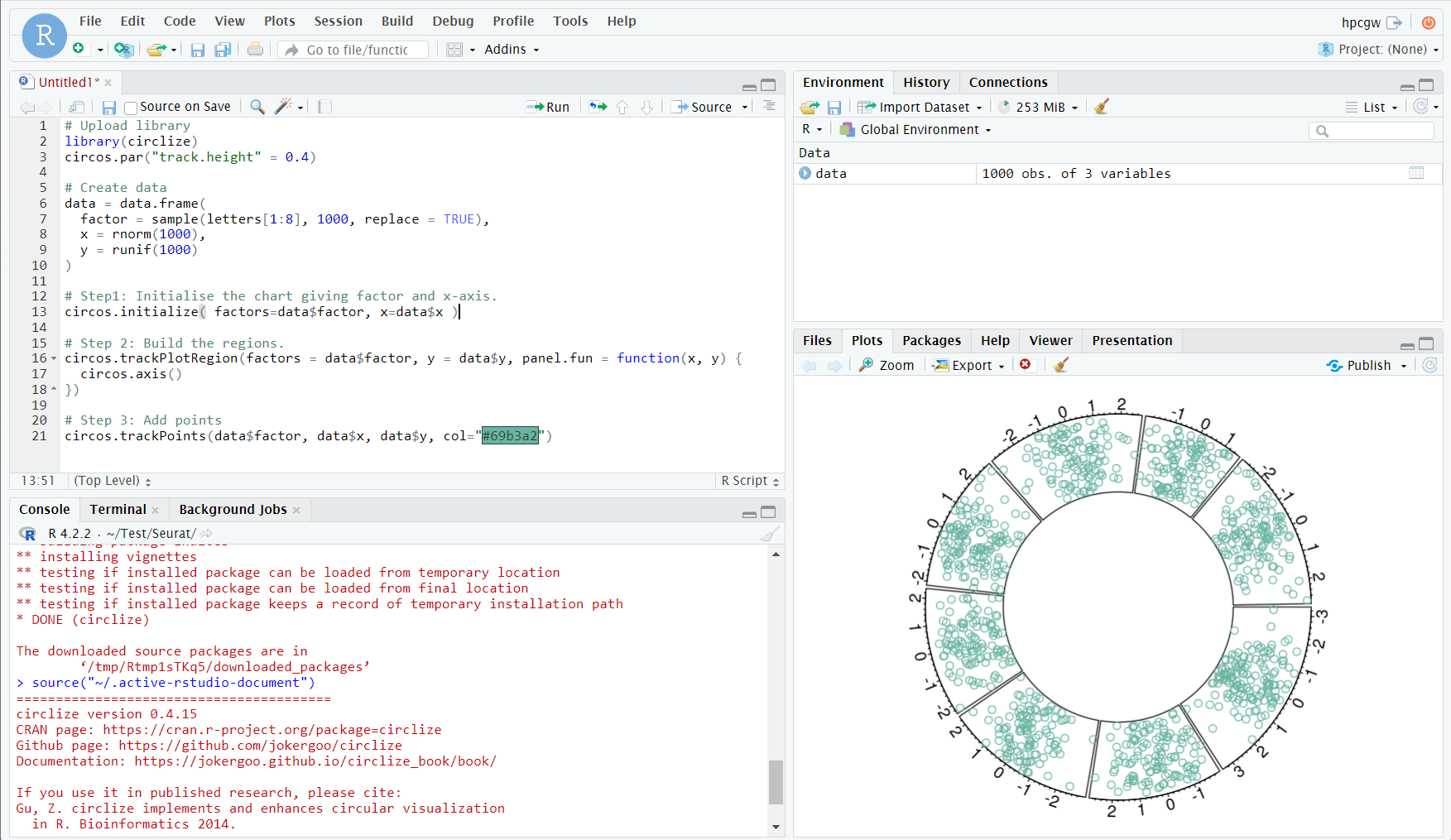# RStudio¶

## 简介¶

RStudio是一个集成开发环境，主要支持R编程语言，专用于统计计算和图形。它包括一个控制台，支持代码执行的语法编辑器，以及用于绘制，调试和管理工作区的工具。

## 可用的版本¶

R版本

RStudio版本

4.2.2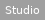2022.12.0

4.1.32022.02.1

4.0.21.2.5042

3.6.31.2.5042

## 如何使用¶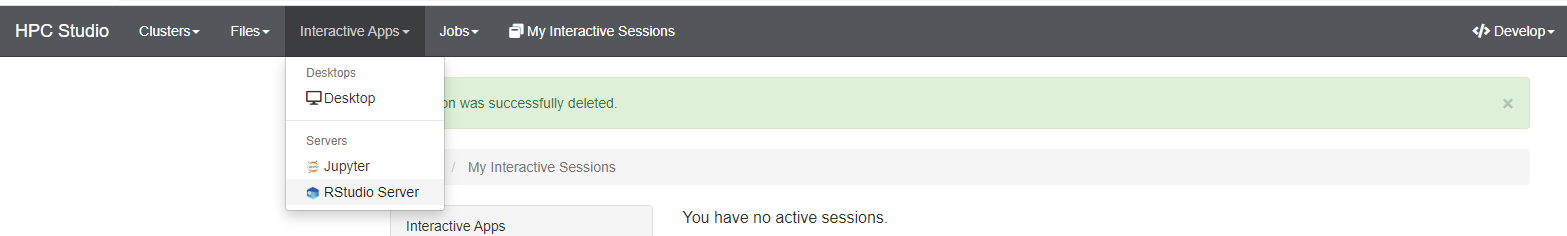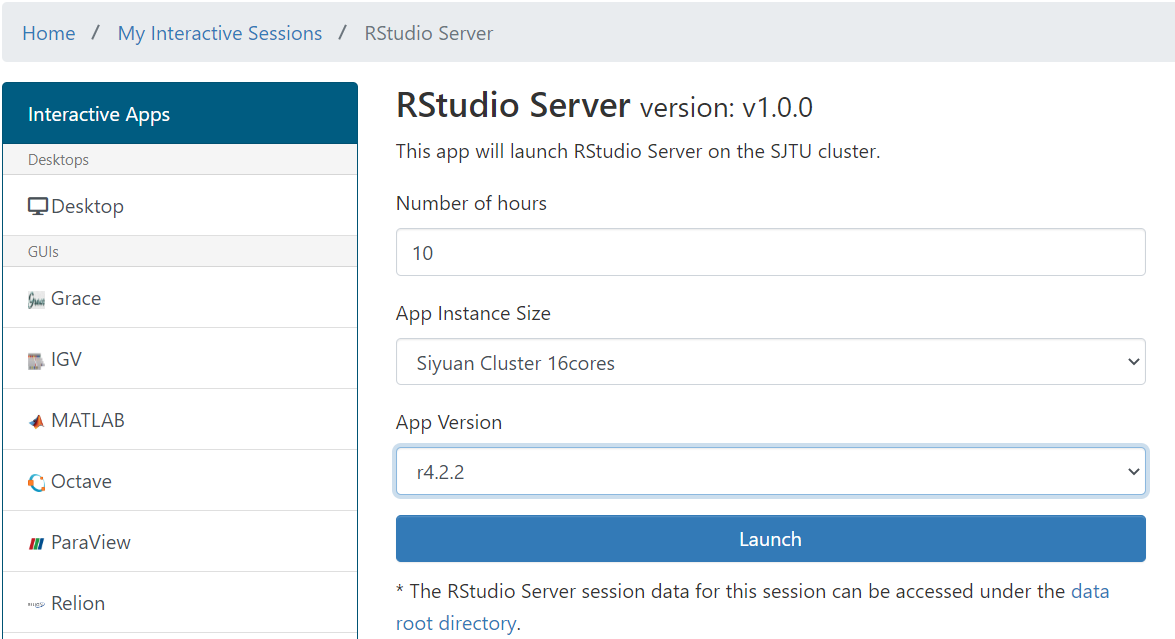Tip

π2.0 集群和思源一号的数据不互通，注意区分。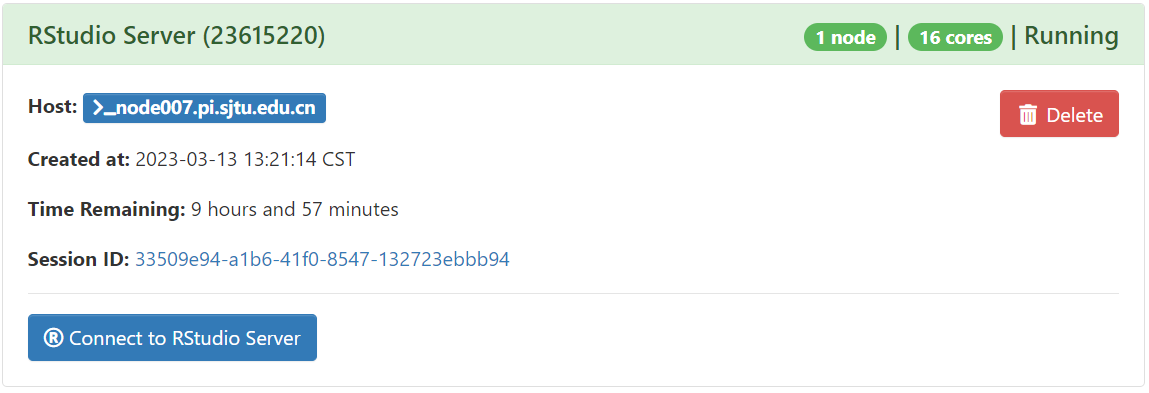## 运行示例¶

``````# Upload library
library(circlize)
circos.par("track.height" = 0.4)

# Create data
data = data.frame(
factor = sample(letters[1:8], 1000, replace = TRUE),
x = rnorm(1000),
y = runif(1000)
)

# Step1: Initialise the chart giving factor and x-axis.
circos.initialize( factors=data\$factor, x=data\$x )

# Step 2: Build the regions.
circos.trackPlotRegion(factors = data\$factor, y = data\$y, panel.fun = function(x, y) {
circos.axis()
})